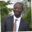Related Tags

javascript
array
concat
communitycreator

# How to find the union of two arrays in JavaScriptAbel Lifaefi Mbula

### Overview

In mathematics, the union of two sets, A and B, is a new set containing all the elements in set A or set B. It is denoted as follows: A U B

### Example

Let's consider three sets: A and B, from which we'll have the third one, C.

• A = {orange, green, blue}
• B = {yellow, white, blue}
• The union C = A U B = {orange, green, blue, yellow, white}.

### Venn diagram

The Venn diagram for the union looks as follows:

The Venn diagram for union

### Code

We know something about a union in mathematics. Now, it's time to practice by implementing it in JavaScript. Considering the above examples, let's create two arrays of color strings that we will be using as a test example.

const arr1 = ['orange', 'green', 'blue']
const arr2 = ['yellow', 'white', 'blue']

Next, let's create the base function for our program.

function union(arr1, arr2) {
const arr3 = []

return arr3
}
Base function

The function union() accepts two parameters, arr1 and arr2, and returns a third array, arr3.

Let's think about how to find the union of arr1 and arr2. I can see three possible options that we can try: concat(), spread operator, or spread operator with Set.

### concat()

concat() is used to merge arrays. In our situation, this method would help us if we had sets with unique values. If not, we will have a merged array with duplicate values, which is not the union we are looking for. Let's try it out.

function union(arr1, arr2) {
const arr3 = arr1.concat(arr2)

return arr3
}

console.log(union(['orange', 'green', 'blue'], ['yellow', 'white', 'blue']))
concat()

If you run this function, you'll have this output [ 'orange', 'green', 'blue', 'yellow', 'white', 'blue' ]. As you can see, the string blue is repeated twice.

### Spread operator (...)

We use the spread operator (...) when we want to include all elements from an array in a list. As with the concat() method, this operator is helpful for us if we have arrays made of unique values.

function union(arr1, arr2) {
const arr3 = [ ...arr1, ...arr2]

return arr3
}

console.log(union(['orange', 'green', 'blue'], ['yellow', 'white', 'blue']))

As in the concat() method above, we have duplicate values.

### Spread operator and Set()

Set() allows us to store unique values. Here's how we can use it with the spread operator and fix the issue we encountered using other methods.

function union(arr1, arr2) {
const arr3 = [ ...new Set([...arr1, ...arr2])]

return arr3
}

console.log(union(['orange', 'green', 'blue'], ['yellow', 'white', 'blue']))

This last solution is the one that fits our case. The output is an array made of unique values.

Thank you for learning with me!

RELATED TAGS

javascript
array
concat
communitycreator

CONTRIBUTORAbel Lifaefi Mbula
RELATED COURSES

View all Courses

Keep Exploring

Learn in-demand tech skills in half the time